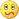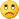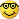# Martingale EA - page 48420

right; and thinking about it again, maybe I had it wrong for the combined risk...

... and maybe reducing the lot size is the wrong conclusion.

I'm confusing myself....[Deleted]

kayvan....thank your lucky stars ....there is a recent poster here that in my opinion is the best in the business of coding...

just be quiet and let them work...kayvan...good things are coming...trust me

ES420

The amount of risk must stay s0*v0+s1*v1, but the probability of risk occurring relates to s0-N for v0 and s1 for v1; but I had hoped not to have to articulate probabilityThus, I think the combined risk at trade 2 is: s0*v0+s1*v1, and this (of course) can be made less or more by varying s1 and/or v1.

If the trades are closed together, you essentially have p0+s0 = p1+s1, which is equivalent to s1 = s0+(p0-p1), and with N the pips difference p1-p0, you get the risk s0*v0+(s0-N)*v1

So in fact, if you want a risk doubling by the second trade, you'd make s0*v0=(s0-N)*v1, or v1 = vo*(s0/(s0-N))

which says that v1 is an amount larger than v0 (when N>0, i.e. new trade at higher price)[Deleted]

ralph,

Can you take it in plain speak...for us not so...ummmmm...nimble minded...

ES420

Ok; I've read in on the thread more now, and realize the approach here is slightly different from what I first thought, but fortunately mathematics stay the same. There's only a difference on what's constant and what's variable.

So, if I understood it correctly, the EA manages a constant risk amount, R, across a series of trades. It would then be of interest to know how the effective stoplevel changes when a new trade is made. Well, I thought so, at least.Just to short-cut: for the second trade, you end up with a mathematical equation that involves 5 variables: the number of pips, P, that price has moved in favourable direction, the first lot size, v0, and first stop-level s0, and the second lots size, v1, and stoplevel s1. These would be related as:

s1/(s0+P) = v0/(v0+v1)

In other words, since P, s0, and v0 are given, you can choose s1 by varying v1. (The first stoplevel is derived from the desired constant risk R and the first lot size v0; the second stoplevel is derivable in this way because the total risk level should be constant, R)

The takeprofit reasoning is similar, though with a sign difference, and you arrive at: t1/(t0-P) = v0/(v0+v1)

By this reasoning (if I've done it right), you arrive at a risk ratio equation that looks like: t1/s1 = (t0-P)/(s0+P)

That is, you should consider adding short on short, or long on long for a negative P (i.e., when the first trade is negative), because that leads to higher takeprofit over stoploss ratio. That's regardless of lot size, which "merely" affects which the resulting takeprofit and stoploss are.

Doing the opposite, i.e., adding short on short or long on long, when the first trade is positive does the opposite, i.e. it reduces the TP/SL ratio.

It may be worth for someone to verify these equations, because it is a fairly strong result.[Deleted]

Ralph,

But if the variable is the length of the candlestick, wouldn't that random introduction cause the answer to the equation simply be a balancing reaction with lotsize?

So your theory is to balance range with lotsize?

ES420

Yes, I think so. If you add on a smaller lot size, v1, the resulting effective stoploss, s1, is larger than if you add on a larger lot size. And that's the additional consideration for the EA: which effective stoploss do you want or need?

At negative P, the new effective stoploss is successively smaller than the one before, and always more than P pips smaller. If then v1 (the new lot size) is large, then s1 (the new effective stoploss) gets really small.

edit: that's where you might have to start considering the price movement probabilities.

edit again: hmm, so what about long on short or short on long?[Deleted]

Well Ralph...Averaging down or Pyramiding in...would be the question for kayvan....I am not the author of this EA...

as both answers are not wrong or correct...just a method for the EA to take...

Thank you for your time thus far.

ES[Deleted]

What interested me with the kayvan method, was its look at the previous candlestick...perhaps looking at the last two candlesticks could have a smoothing effect if the EA were not hardcoded for TF.

ES[Deleted]

looking back too far...may detract from the mathematical progression that this EA method wants to take.

This EA is not a statistical trader and should not use too much weighting on probability prediction or trend prediction...simply using the candlestick (s) breaches will accomplish some of that for the purposes of lotsize choice.

I believe that the kayvan EA is more weighted in the mathematical progressive reaction to the market....a dangerous approach in a long term trend direction...but hmmmm...not really as that would be what is needed to take the trade out...must think...pause...scratching head...

ES

Late Edit:Range or intermittent Range Kills